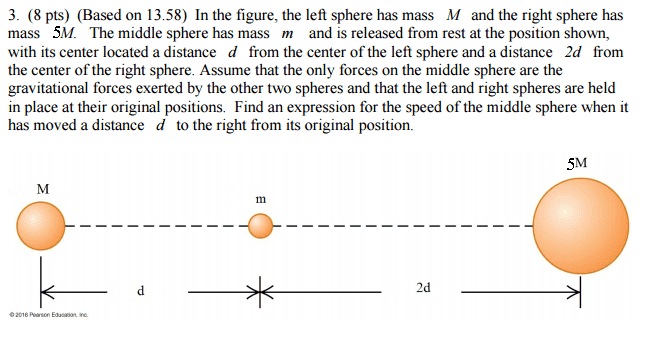# Gravitational Potential Energy of a Sphere

• reminiscent

## Homework Statement## Homework Equations

ΔPE = G × M₁ × M₂ (1/Ri - 1/Rf)
where
G = gravitational constant
M₁ = mass of one object
M₂ = mass of the other object
Ri = initial distance
Rf = final distance
ΔPE = -ΔKE

## The Attempt at a Solution

My solution is v = 2√(GM/d). I am making sure it is correct.

TSny said:
ΔPE = G × M₁ × M₂ (1/Ri - 1/Rf)
ΔPE 5M= G × 5M × m (1/2d - 1/d) = -5GMm/2d
ΔPE M= G × M × m (1/d - 1/2d) = GMm/2d
ΔPE total = ΔPE 5M + ΔPE M = -2GMm/d

ΔPE = -ΔKE = 2GMm/d
(1/2)mv2 = 2GMm/d
v = 2√(GM/d)

reminiscent said:
ΔPE = G × M₁ × M₂ (1/Ri - 1/Rf)
ΔPE 5M= G × 5M × m (1/2d - 1/d) = -5GMm/2d
ΔPE M= G × M × m (1/d - 1/2d) = GMm/2d
ΔPE total = ΔPE 5M + ΔPE M = -2GMm/d

ΔPE = -ΔKE = 2GMm/d
(1/2)mv2 = 2GMm/d
v = 2√(GM/d)
Looks good.